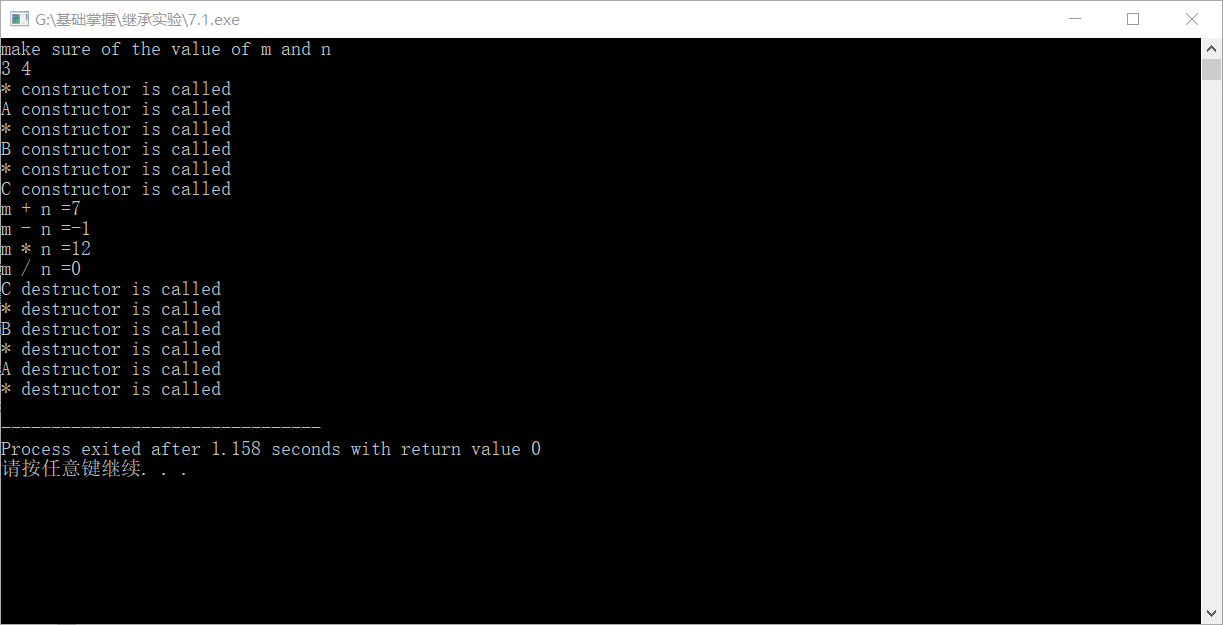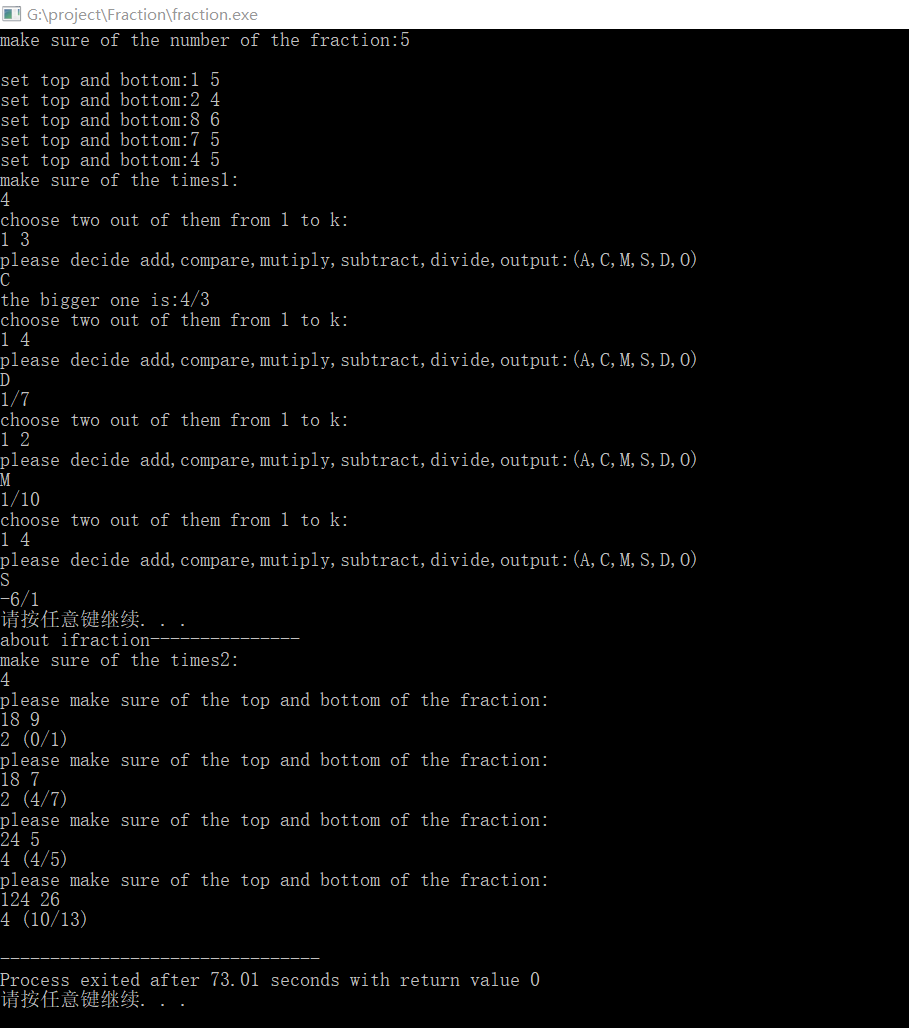# 实验6，继承

//7.1#include<iostream>
using namespace std;
class Total{
public:
Total(int i,int j){
cout<<"* constructor is called"<<endl;
n=i;
m=j;
}
Total(){
cout<<"* constructor is called"<<endl;
n=0;
m=0;
}
Total(Total &total){
cout<<"* copy constructor is called"<<endl;
n=total.n;
m=total.m;
}
return n+m;
}
~Total() {cout<<"* destructor is called"<<endl;}
private:
int n;
int m;
};
class A: public Total{
private:
int n;
int m;
public:
A(int a,int b): Total(a,b),n(a),m(b) {cout<<"A constructor is called"<<endl;};
A():Total(0,0),n(0),m(0) {cout<<"A constructor is called"<<endl;};
int subtract(){
return n-m;
}
~A(){cout<<"A destructor is called"<<endl;}
};
class B: public Total{
private:
int n;
int m;
public:
B(int a,int b): Total(a,b),n(a),m(b) {cout<<"B constructor is called"<<endl;};
B():Total(0,0),n(0),m(0) {cout<<"B constructor is called"<<endl;};
int multiply(){
return n*m;
}
~B(){cout<<"B destructor is called"<<endl;}
};
class C: public Total{
private:
int n;
int m;
public:
C(int a,int b): Total(a,b),n(a),m(b) {cout<<"C constructor is called"<<endl;};
C():Total(0,0),n(0),m(0) {cout<<"C constructor is called"<<endl;};
int divide(){
return n/m;
}
~C(){cout<<"C destructor is called"<<endl;}
};
int main()
{
int m,n;
cout<<"make sure of the value of m and n"<<endl;
cin>>m>>n;
A a(m,n);
B b(m,n);
C c(m,n);
cout<<"m + n =";
cout<<"m - n ="<<a.subtract()<<endl;
cout<<"m * n ="<<b.multiply()<<endl;
cout<<"m / n ="<<c.divide()<<endl;
return 0;
}//7.2#include<iostream>
using namespace std;
class vehicle{
public:
vehicle(int i,int j) : maxspeed(i) , weight(j){cout<<"vehicle's constructor is called"<<endl;}
vehicle() : maxspeed(0) , weight(0){cout<<"vehicle's constructor is called"<<endl;}
void initvehicle(int n,int m){maxspeed=n;weight=n;}
void run(){
cout<<"the vehicle's weight is "<<weight<<endl;
cout<<"the vehicle's speed is "<<maxspeed<<endl;
}
void stop(){
cout<<"the vehicle has stopped "<<endl;
}
~vehicle(){cout<<"vehicle's destructor is called"<<endl;}
protected:
int maxspeed;
int weight;
};
class bicycle:virtual public vehicle{
public:
bicycle(int i,int j,int k):vehicle(i,j),height(k){cout<<"the vehicle(bicycle)'s constructor is called"<<endl;}
bicycle():vehicle(0,0),height(0){cout<<"the vehicle(bicycle)'s constructor is called"<<endl;}
void initbicycle(int n){height=n;}
~bicycle(){cout<<"vehicle(bicycle)'s destructor is called"<<endl;}
protected:
int height;
};
class motorcar:virtual public vehicle{
public:
motorcar(int i,int j,int k):vehicle(i,j),seatnum(k){cout<<"the vehicle(motorcar)'s constructor is called"<<endl;}
motorcar():vehicle(0,0),seatnum(0){cout<<"the vehicle(motorcar)'s constructor is called"<<endl;}
void initmotorcar(int n){seatnum=n;}
~motorcar(){cout<<"vehicle(motorcar)'s destructor is called"<<endl;}
protected:
int seatnum;
};
class motorcycle:public bicycle,public motorcar{
public:
motorcycle(int i,int j,int k,int p):vehicle(i,j),bicycle(i,j,k),motorcar(i,j,p){cout<<"the vehicle(motorcycle)'s constructor is called"<<endl;}
motorcycle():vehicle(),bicycle(),motorcar(){cout<<"the vehicle(motorcycle)'s constructor is called"<<endl;}
~motorcycle(){cout<<"vehicle(motorcycle)'s destructor is called"<<endl;}
};
int main(){
int m,n,p,q;
cin>>m>>n;
cin>>p>>q;
motorcycle mc(m,n,p,q);
system("pause");
mc.run();
system("pause");
mc.stop();
system("pause");
return 0;
}//7.3 代码大部分见上次fraction类

//main中添加代码
system("pause");
cout<<"make sure of the times2:"<<endl;
int times2;
cin>>times2;
while(times2--){
int p,q;
cout<<"please make sure of the top and bottom of the fraction: "<<endl;
cin>>p>>q;
iFraction ifraction(p,q);
ifraction.legal();
convertF(ifraction);
ifraction.print();

//iFraction类
class iFraction :public Fraction{
public:
iFraction(int n,int m):Fraction(n,m),wholepart(0),fractionparttop(0),fractionpartbottom(0){}
~iFraction(){}
friend void convertF(iFraction &A);
void print();
private:
int wholepart;
int fractionparttop;
int fractionpartbottom;
};
//ifraction 实现
#include<iostream>
#include"ifraction.h"
using namespace std;
void convertF(iFraction &A){
int i;
i=A.top;
int j;
j=A.bottom;
if(i>0){
A.wholepart=i/j;
A.fractionparttop=i-A.wholepart*j;
A.fractionpartbottom=j;
}
if(i<0){
i=-1*i;
A.wholepart=i/j;
A.fractionparttop=i-A.wholepart*j;
A.fractionpartbottom=j;
A.wholepart=-1*A.wholepart;
}
}

void iFraction::print(){
if(wholepart!=0)
cout<<wholepart<<" ("<<fractionparttop<<"/"<<fractionpartbottom<<")"<<endl;
else
cout<<fractionparttop<<"/"<<fractionpartbottom<<endl;
}posted @ 2018-06-07 00:02  tacore  阅读(99)  评论(0编辑  收藏  举报